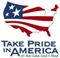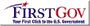Open-File Report 2006-1312

U.S. GEOLOGICAL SURVEY
Open-File Report 2006-1312

# Evapotranspiration Estimates

Evapotranspiration is a process by which water from the Earth’s surface is transferred to the atmosphere. The transfer requires that water change from a liquid to a vapor state, which consumes energy. As a result, any change in the rate of water loss by ET is reflected by a change in energy. This relation between water loss and energy consumption is the basis for many of the methods used to estimate ET. Evapotranspiration, as used in this report, includes evaporation from the soil surface, transpiration from plants, and sublimation from snowpack.

Energy at the surface of the Earth can be described as a budget, in which a balance is sought between the incoming and outgoing energy components. The available incoming or net radiation is the energy provided by the sun minus the radiation that is reflected back. This net radiation is equal to the energy used to heat the soil (soil heat flux), plus the energy used to heat the air (sensible heat flux), plus the energy used to evaporate water (latent heat flux). Terms related to the flux of energy can be expressed mathematically as:

Rn = Gs + H + λE ,      (1)

where

 Rn is net radiation, in Watts per square meter, Gs is soil heat flux, in Watts per square meter, H is sensible heat flux, in Watts per square meter, and λE is latent heat flux, in Watts per square meter.

In this study, the Bowen ratio (Bowen, 1926) and eddy covariance (Stull, 1988) methods were applied to calculate ET rates. The Bowen ratio method requires measurements of air temperature and relative humidity at two heights above the ground. These measurements are used to calculate air-temperature and vapor-pressure gradients between the two sample heights (fig. 8A, table 1). The Bowen ratio works on the principle that the temperature and vapor-pressure gradients and the available energy regulate the latent heat flux. The eddy covariance method requires measurements of vertical wind speed and fluctuations in water vapor sampled at 0.1-second intervals using a three-dimensional sonic anemometer and a krypton hygrometer, respectively (fig. 8B, table 1). The eddy covariance method works on the principle that water vapor and heat are transported through the air by turbulence and that estimates of latent heat flux can be calculated by measuring changes in vertical wind speed, air temperature, and water vapor during short intervals of time. Corrections for temperature-induced fluctuations in air density (Webb and others, 1980) and for the sensitivity of the krypton hygrometer to oxygen (Tanner and Greene, 1989) were used to estimate latent heat flux. More detailed discussions on the Bowen ratio and eddy covariance methods are given in Laczniak and others (1999), and DeMeo and others (2003), respectively.

Numerous days of Bowen ratio data were missing at both sites due to the effects of adverse weather conditions and instrumentation failure. In 2003, the tree site was destroyed in a wind storm and no data were collected from January 4 to May 15. A more complete record of ET from the eddy covariance instrumentation was made available due to the robustness of the equipment. Data collection periods from each site, when sufficient data were available to calculate daily ET, are listed in table 2.

Daily ET using the Bowen ratio and eddy covariance methods was determined by summing 20-minute average ET rates for a 24-hour period. Calculated daily ET from 2002 to 2005 for each site is shown in figure 9. Daily ET was summed to calculate monthly and annual ET. Table 3 lists the monthly ET collected at each site during the period of record. Annual ET for the brush site using the eddy covariance method (2002–04) ranged from 243.6 to 347.5 mm. Annual ET for the tree site using the eddy covariance method (2002 and 2004) ranged from 315.0 to 313.0 mm.U.S. Department of the Interior | U.S. Geological Survey